1605214440

# Lucht Probst Associates Takes Advantage of Azure Innovation and Security

Lucht Probst Associates (LPA), a leader in capital technology, helps transform and digitize players in the ecosystem of capital markets, from local authorities to banks and insurers. Their migration project was supported with the complete set of Azure Migration Program offerings.

Please note: some of the footage used in this video was pre-recorded prior to the COVID-19 pandemic.

#azure #security #programming #developer

## Buddha Community1672928580

## How to Bash Read Command

Bash has no built-in function to take the user’s input from the terminal. The read command of Bash is used to take the user’s input from the terminal. This command has different options to take an input from the user in different ways. Multiple inputs can be taken using the single read command. Different ways of using this command in the Bash script are described in this tutorial.

## Syntax

The read command can be used without any argument or option. Many types of options can be used with this command to take the input of the particular data type. It can take more input from the user by defining the multiple variables with this command.

## Some Useful Options of the Read Command

Some options of the read command require an additional parameter to use. The most commonly used options of the read command are mentioned in the following:

## Different Examples of the Read Command

The uses of read command with different options are shown in this part of this tutorial.

Example 1: Using Read Command without Any Option and variable

Create a Bash file with the following script that takes the input from the terminal using the read command without any option and variable. If no variable is used with the read command, the input value is stored in the \$REPLY variable. The value of this variable is printed later after taking the input.

``````#!/bin/bash
#Print the prompt message
echo "Enter your favorite color: "
#Take the input
#Print the input value

Output:

The following output appears if the “Blue” value is taken as an input:Example 2: Using Read Command with a Variable

Create a Bash file with the following script that takes the input from the terminal using the read command with a variable. The method of taking the single or multiple variables using a read command is shown in this example. The values of all variables are printed later.

``````#!/bin/bash
#Print the prompt message
echo "Enter the product name: "
#Take the input with a single variable

#Print the prompt message
echo "Enter the color variations of the product: "
#Take three input values in three variables

#Print the input value
echo "The product name is \$item."
#Print the input values
echo "Available colors are \$color1, \$color2, and \$color3."``````

Output:

The following output appears after taking a single input first and three inputs later: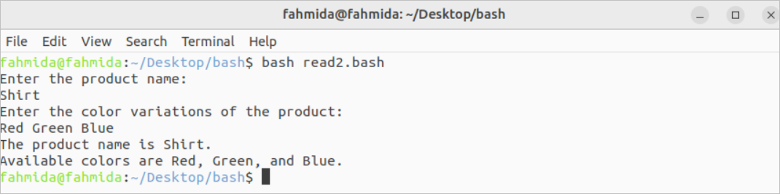Example 3: Using Read Command with -p Option

Create a Bash file with the following script that takes the input from the terminal using the read command with a variable and the -p option. The input value is printed later.

``````#!/bin/bash
#Take the input with the prompt message
read -p "Enter the book name: " book
#Print the input value
echo "Book name: \$book"``````

Output:

The following output appears after taking the input: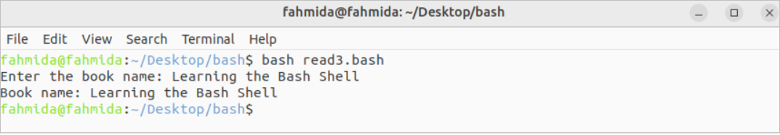Example 4: Using Read Command with -s Option

Create a Bash file with the following script that takes the input from the terminal using the read command with a variable and the -s option. The input value of the password will not be displayed for the -s option. The input values are checked later for authentication. A success or failure message is also printed.

``````#!/bin/bash
#Take the input with the prompt message
#Take the secret input with the prompt message

echo ""

#Check the email and password for authentication
then
#Print the success message
echo "Authenticated."
else
#Print the failure message
echo "Not authenticated."
fi``````

Output:

The following output appears after taking the valid and invalid input values: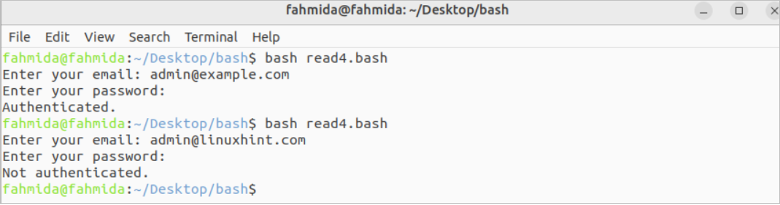Example 5: Using Read Command with -a Option

Create a Bash file with the following script that takes the input from the terminal using the read command with a variable and the -a option. The array values are printed later after taking the input values from the terminal.

``````#!/bin/bash
echo "Enter the country names: "
#Take multiple inputs using an array

echo "Country names are:"
for country in \${countries[@]}
do
echo \$country
done``````

Output:

The following output appears after taking the array values: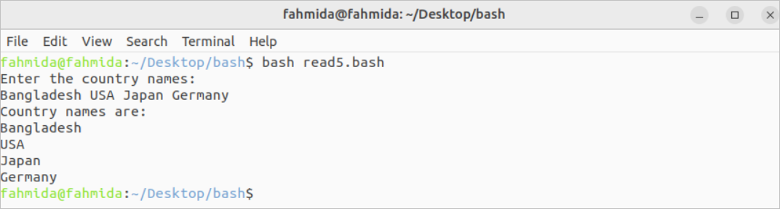Example 6: Using Read Command with -n Option

Create a Bash file with the following script that takes the input from the terminal using the read command with a variable and the -n option.

``````#!/bin/bash
#Print the prompt message
echo "Enter the product code: "
#Take the input of five characters
echo ""
#Print the input value
echo "The product code is \$code"``````

Output:

The following output appears if the “78342” value is taken as input: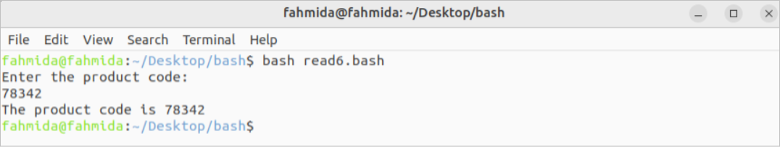Example 7: Using Read Command with -t Option

Create a Bash file with the following script that takes the input from the terminal using the read command with a variable and the -t option.

``````#!/bin/bash
#Print the prompt message
echo -n "Write the result of 10-6: "
#Take the input of five characters

#Check the input value
if [[ \$answer == "4" ]]
then
else
fi``````

Output:

The following output appears after taking the correct and incorrect input values: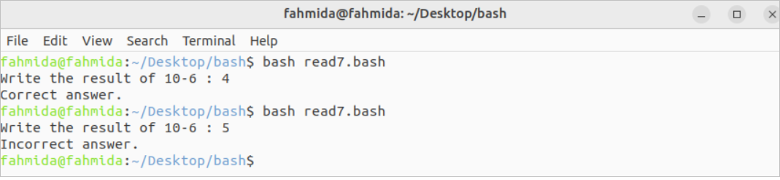## Conclusion

The uses of some useful options of the read command are explained in this tutorial using multiple examples to know the basic uses of the read command.

Original article source at: https://linuxhint.com/

1637595900

## Nesta postagem python, você aprenderá o seguinte:

• O que é uma pesquisa linear?
• Algoritmo de pesquisa linear
• Escreva um programa Python para pesquisa linear usando loop while
• Escreva um programa Python para pesquisa linear usando o loop for
• Pesquisa linear no programa Python usando recursão

### O que é uma pesquisa linear?

Em primeiro lugar, uma pesquisa linear, também conhecida como pesquisa sequencial, este método é usado para localizar um elemento dentro de uma lista ou array. Ele verifica cada elemento da lista um por um / sequencialmente até que uma correspondência seja encontrada ou toda a lista tenha sido pesquisada.

### Algoritmo de pesquisa linear

Implemente a pesquisa linear seguindo as etapas abaixo:

• Percorra a lista / array usando um loop.
• Em cada iteração, associe o `target` valor ao  valor fornecido da lista / matriz.
• Se os valores corresponderem, retorne o índice atual da lista / matriz.
• Caso contrário, vá para o próximo elemento de array / lista.
• Se nenhuma correspondência for encontrada, retorne  `-1`.

### Escreva um programa Python para pesquisa linear usando loop while

``````# python program for linear search using while loop

#define list
lst = []

#take input list size
num = int(input("Enter size of list :- "))

for n in range(num):
#append element in list/array
numbers = int(input("Enter the array of %d element :- " %n))
lst.append(numbers)

#take input number to be find in list
x = int(input("Enter number to search in list :- "))

i = 0
flag = False

while i < len(lst):
if lst[i] == x:
flag = True
break

i = i + 1

if flag == 1:
print('{} was found at index {}.'.format(x, i))
else:
``````

Depois de executar o programa, a saída será:

``````Enter size of list :-  5
Enter the array of 0 element :-  10
Enter the array of 1 element :-  23
Enter the array of 2 element :-  56
Enter the array of 3 element :-  89
Enter the array of 4 element :-  200
Enter number to search in list :-  89
89 was found at index 3.
``````

### Escreva um programa Python para pesquisa linear usando o loop for

``````# python program for linear search using for loop

#define list
lst = []

#take input list size
num = int(input("Enter size of list :- "))

for n in range(num):
#append element in list/array
numbers = int(input("Enter the array of %d element :- " %n))
lst.append(numbers)

#take input number to be find in list
x = int(input("Enter number to search in list :- "))

i = 0
flag = False

for i in range(len(lst)):
if lst[i] == x:
flag = True
break

if flag == 1:
print('{} was found at index {}.'.format(x, i))
else:

``````

Depois de executar o programa, a saída será:

``````Enter size of list :-  6
Enter the array of 0 element :-  25
Enter the array of 1 element :-  50
Enter the array of 2 element :-  100
Enter the array of 3 element :-  200
Enter the array of 4 element :-  250
Enter the array of 5 element :-  650
Enter number to search in list :-  200
200 was found at index 3.

``````

### Pesquisa linear no programa Python usando recursão

``````# python program for linear search using for loop

#define list
lst = []

#take input list size
num = int(input("Enter size of list :- "))

for n in range(num):
#append element in list/array
numbers = int(input("Enter the array of %d element :- " %n))
lst.append(numbers)

#take input number to be find in list
x = int(input("Enter number to search in list :- "))

# Recursive function to linear search x in arr[l..r]
def recLinearSearch( arr, l, r, x):
if r < l:
return -1
if arr[l] == x:
return l
if arr[r] == x:
return r
return recLinearSearch(arr, l+1, r-1, x)

res = recLinearSearch(lst, 0, len(lst)-1, x)

if res != -1:
print('{} was found at index {}.'.format(x, res))
else:

``````

Depois de executar o programa, a saída será:

``````Enter size of list :-  5
Enter the array of 0 element :-  14
Enter the array of 1 element :-  25
Enter the array of 2 element :-  63
Enter the array of 3 element :-  42
Enter the array of 4 element :-  78
Enter number to search in list :-  78
78 was found at index 4.``````1637592180

## В этом посте на Python вы узнаете следующее:

• Что такое линейный поиск?
• Алгоритм линейного поиска
• Напишите программу на Python для линейного поиска с использованием цикла while
• Напишите программу на Python для линейного поиска с использованием цикла For
• Линейный поиск в программе Python с использованием рекурсии

### Что такое линейный поиск?

Прежде всего, линейный поиск, также известный как последовательный поиск, этот метод используется для поиска элемента в списке или массиве. Он проверяет каждый элемент списка один за другим / последовательно, пока не будет найдено совпадение или пока не будет выполнен поиск по всему списку.

### Алгоритм линейного поиска

Реализуйте линейный поиск, выполнив следующие шаги:

• Просмотрите список / массив, используя цикл.
• На каждой итерации связывайте  `target` значение с заданным значением списка / массива.
• Если значения совпадают, вернуть текущий индекс списка / массива.
• В противном случае перейдите к следующему элементу массива / списка.
• Если совпадений не найдено, вернитесь  `-1`.

### Напишите программу на Python для линейного поиска с использованием цикла while

``````# python program for linear search using while loop

#define list
lst = []

#take input list size
num = int(input("Enter size of list :- "))

for n in range(num):
#append element in list/array
numbers = int(input("Enter the array of %d element :- " %n))
lst.append(numbers)

#take input number to be find in list
x = int(input("Enter number to search in list :- "))

i = 0
flag = False

while i < len(lst):
if lst[i] == x:
flag = True
break

i = i + 1

if flag == 1:
print('{} was found at index {}.'.format(x, i))
else:
``````

После выполнения программы вывод будет:

``````Enter size of list :-  5
Enter the array of 0 element :-  10
Enter the array of 1 element :-  23
Enter the array of 2 element :-  56
Enter the array of 3 element :-  89
Enter the array of 4 element :-  200
Enter number to search in list :-  89
89 was found at index 3.
``````

### Напишите программу на Python для линейного поиска с использованием цикла For

``````# python program for linear search using for loop

#define list
lst = []

#take input list size
num = int(input("Enter size of list :- "))

for n in range(num):
#append element in list/array
numbers = int(input("Enter the array of %d element :- " %n))
lst.append(numbers)

#take input number to be find in list
x = int(input("Enter number to search in list :- "))

i = 0
flag = False

for i in range(len(lst)):
if lst[i] == x:
flag = True
break

if flag == 1:
print('{} was found at index {}.'.format(x, i))
else:

``````

После выполнения программы вывод будет:

``````Enter size of list :-  6
Enter the array of 0 element :-  25
Enter the array of 1 element :-  50
Enter the array of 2 element :-  100
Enter the array of 3 element :-  200
Enter the array of 4 element :-  250
Enter the array of 5 element :-  650
Enter number to search in list :-  200
200 was found at index 3.

``````

### Линейный поиск в программе Python с использованием рекурсии

``````# python program for linear search using for loop

#define list
lst = []

#take input list size
num = int(input("Enter size of list :- "))

for n in range(num):
#append element in list/array
numbers = int(input("Enter the array of %d element :- " %n))
lst.append(numbers)

#take input number to be find in list
x = int(input("Enter number to search in list :- "))

# Recursive function to linear search x in arr[l..r]
def recLinearSearch( arr, l, r, x):
if r < l:
return -1
if arr[l] == x:
return l
if arr[r] == x:
return r
return recLinearSearch(arr, l+1, r-1, x)

res = recLinearSearch(lst, 0, len(lst)-1, x)

if res != -1:
print('{} was found at index {}.'.format(x, res))
else:

``````

После выполнения программы вывод будет:

``````Enter size of list :-  5
Enter the array of 0 element :-  14
Enter the array of 1 element :-  25
Enter the array of 2 element :-  63
Enter the array of 3 element :-  42
Enter the array of 4 element :-  78
Enter number to search in list :-  78
78 was found at index 4.``````

1637570520

## Dans cet article Python, vous apprendrez ce qui suit :

• Qu'est-ce qu'une recherche linéaire ?
• Algorithme de recherche linéaire
• Écrire un programme Python pour la recherche linéaire à l'aide de la boucle While
• Écrire un programme Python pour la recherche linéaire à l'aide de la boucle For
• Recherche linéaire dans le programme Python à l'aide de la récursion

### Qu'est-ce qu'une recherche linéaire ?

Tout d'abord, une recherche linéaire, également appelée recherche séquentielle, cette méthode est utilisée pour rechercher un élément dans une liste ou un tableau. Il vérifie chaque élément de la liste un par un / séquentiellement jusqu'à ce qu'une correspondance soit trouvée ou que toute la liste ait été recherchée.

### Algorithme de recherche linéaire

Implémentez la recherche linéaire en suivant les étapes ci-dessous :

• Parcourez la liste/le tableau à l'aide d'une boucle.
• À chaque itération, associez la  `target` valeur à la valeur donnée de la liste/du tableau.
• Si les valeurs correspondent, renvoie l'index actuel de la liste/du tableau.
• Sinon, passez à l'élément de tableau/liste suivant.
• Si aucune correspondance n'est trouvée, retournez  `-1`.

### Écrire un programme Python pour la recherche linéaire à l'aide de la boucle While

``````# python program for linear search using while loop

#define list
lst = []

#take input list size
num = int(input("Enter size of list :- "))

for n in range(num):
#append element in list/array
numbers = int(input("Enter the array of %d element :- " %n))
lst.append(numbers)

#take input number to be find in list
x = int(input("Enter number to search in list :- "))

i = 0
flag = False

while i < len(lst):
if lst[i] == x:
flag = True
break

i = i + 1

if flag == 1:
print('{} was found at index {}.'.format(x, i))
else:
``````

Après l'exécution du programme, la sortie sera :

``````Enter size of list :-  5
Enter the array of 0 element :-  10
Enter the array of 1 element :-  23
Enter the array of 2 element :-  56
Enter the array of 3 element :-  89
Enter the array of 4 element :-  200
Enter number to search in list :-  89
89 was found at index 3.
``````

### Écrire un programme Python pour la recherche linéaire à l'aide de la boucle For

``````# python program for linear search using for loop

#define list
lst = []

#take input list size
num = int(input("Enter size of list :- "))

for n in range(num):
#append element in list/array
numbers = int(input("Enter the array of %d element :- " %n))
lst.append(numbers)

#take input number to be find in list
x = int(input("Enter number to search in list :- "))

i = 0
flag = False

for i in range(len(lst)):
if lst[i] == x:
flag = True
break

if flag == 1:
print('{} was found at index {}.'.format(x, i))
else:

``````

Après l'exécution du programme, la sortie sera :

``````Enter size of list :-  6
Enter the array of 0 element :-  25
Enter the array of 1 element :-  50
Enter the array of 2 element :-  100
Enter the array of 3 element :-  200
Enter the array of 4 element :-  250
Enter the array of 5 element :-  650
Enter number to search in list :-  200
200 was found at index 3.

``````

### Recherche linéaire dans le programme Python à l'aide de la récursion

``````# python program for linear search using for loop

#define list
lst = []

#take input list size
num = int(input("Enter size of list :- "))

for n in range(num):
#append element in list/array
numbers = int(input("Enter the array of %d element :- " %n))
lst.append(numbers)

#take input number to be find in list
x = int(input("Enter number to search in list :- "))

# Recursive function to linear search x in arr[l..r]
def recLinearSearch( arr, l, r, x):
if r < l:
return -1
if arr[l] == x:
return l
if arr[r] == x:
return r
return recLinearSearch(arr, l+1, r-1, x)

res = recLinearSearch(lst, 0, len(lst)-1, x)

if res != -1:
print('{} was found at index {}.'.format(x, res))
else:

``````

Après l'exécution du programme, la sortie sera :

``````Enter size of list :-  5
Enter the array of 0 element :-  14
Enter the array of 1 element :-  25
Enter the array of 2 element :-  63
Enter the array of 3 element :-  42
Enter the array of 4 element :-  78
Enter number to search in list :-  78
78 was found at index 4.``````

1637566860

## En esta publicación de Python, aprenderá lo siguiente:

• ¿Qué es una búsqueda lineal?
• Algoritmo de búsqueda lineal
• Escriba un programa Python para búsqueda lineal usando While Loop
• Escriba un programa Python para búsqueda lineal usando For Loop
• Búsqueda lineal en el programa Python usando recursividad

### ¿Qué es una búsqueda lineal?

En primer lugar, una búsqueda lineal, también conocida como búsqueda secuencial, este método se utiliza para encontrar un elemento dentro de una lista o matriz. Comprueba cada elemento de la lista uno por uno / secuencialmente hasta que se encuentra una coincidencia o se ha buscado en toda la lista.

### Algoritmo de búsqueda lineal

Implemente la búsqueda lineal siguiendo los pasos a continuación:

• Recorre la lista / matriz usando un bucle.
• En cada iteración, asocie el  `target` valor con el valor dado de la lista / matriz.
• Si los valores coinciden, devuelve el índice actual de la lista / matriz.
• De lo contrario, continúe con el siguiente elemento de matriz / lista.
• Si no se encuentra ninguna coincidencia, regrese  `-1`.

### Escriba un programa Python para búsqueda lineal usando While Loop

``````# python program for linear search using while loop

#define list
lst = []

#take input list size
num = int(input("Enter size of list :- "))

for n in range(num):
#append element in list/array
numbers = int(input("Enter the array of %d element :- " %n))
lst.append(numbers)

#take input number to be find in list
x = int(input("Enter number to search in list :- "))

i = 0
flag = False

while i < len(lst):
if lst[i] == x:
flag = True
break

i = i + 1

if flag == 1:
print('{} was found at index {}.'.format(x, i))
else:
``````

Después de ejecutar el programa, la salida será:

``````Enter size of list :-  5
Enter the array of 0 element :-  10
Enter the array of 1 element :-  23
Enter the array of 2 element :-  56
Enter the array of 3 element :-  89
Enter the array of 4 element :-  200
Enter number to search in list :-  89
89 was found at index 3.
``````

### Escriba un programa Python para búsqueda lineal usando For Loop

``````# python program for linear search using for loop

#define list
lst = []

#take input list size
num = int(input("Enter size of list :- "))

for n in range(num):
#append element in list/array
numbers = int(input("Enter the array of %d element :- " %n))
lst.append(numbers)

#take input number to be find in list
x = int(input("Enter number to search in list :- "))

i = 0
flag = False

for i in range(len(lst)):
if lst[i] == x:
flag = True
break

if flag == 1:
print('{} was found at index {}.'.format(x, i))
else:

``````

Después de ejecutar el programa, la salida será:

``````Enter size of list :-  6
Enter the array of 0 element :-  25
Enter the array of 1 element :-  50
Enter the array of 2 element :-  100
Enter the array of 3 element :-  200
Enter the array of 4 element :-  250
Enter the array of 5 element :-  650
Enter number to search in list :-  200
200 was found at index 3.

``````

### Búsqueda lineal en el programa Python usando recursividad

``````# python program for linear search using for loop

#define list
lst = []

#take input list size
num = int(input("Enter size of list :- "))

for n in range(num):
#append element in list/array
numbers = int(input("Enter the array of %d element :- " %n))
lst.append(numbers)

#take input number to be find in list
x = int(input("Enter number to search in list :- "))

# Recursive function to linear search x in arr[l..r]
def recLinearSearch( arr, l, r, x):
if r < l:
return -1
if arr[l] == x:
return l
if arr[r] == x:
return r
return recLinearSearch(arr, l+1, r-1, x)

res = recLinearSearch(lst, 0, len(lst)-1, x)

if res != -1:
print('{} was found at index {}.'.format(x, res))
else:

``````

Después de ejecutar el programa, la salida será:

``````Enter size of list :-  5
Enter the array of 0 element :-  14
Enter the array of 1 element :-  25
Enter the array of 2 element :-  63
Enter the array of 3 element :-  42
Enter the array of 4 element :-  78
Enter number to search in list :-  78
78 was found at index 4.
``````